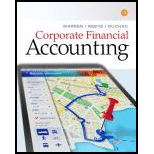# Arch Coal: Times interest earned Arch Coal, Inc. is a major coal mining company in the United States. Condensed income statement information for three recent years follows (in millions): Year 3 Year 2 Year 1 Revenues $2,937$ 3,014 $3,768 Costs and expenses 3,087 3,677 4,52$ Loss from operations $(150)$ (663) $(757) Interest expense (net) 383 375 312 Loss before income taxes$ (533) $(1,038)$(1,069) A. Compute the times interest earned ratio for the three years. (Round to one decimal place.) B. How would you interpret a negative ratio? C. Is the trend improving or deteriorating?### Corporate Financial Accounting

14th Edition
Carl Warren + 2 others
Publisher: Cengage Learning
ISBN: 9781305653535

#### Solutions

Chapter
Section### Corporate Financial Accounting

14th Edition
Carl Warren + 2 others
Publisher: Cengage Learning
ISBN: 9781305653535
Textbook Problem
32 views

## Arch Coal:Times interest earnedArch Coal, Inc. is a major coal mining company in the United States. Condensed income statement information for three recent years follows (in millions): Year 3 Year 2 Year 1 Revenues $2,937$ 3,014 $3,768 Costs and expenses 3,087 3,677 4,52$ Loss from operations $(150)$ (663) $(757) Interest expense (net) 383 375 312 Loss before income taxes$ (533) $(1,038)$(1,069) A. Compute the times interest earned ratio for the three years. (Round to one decimal place.)B. How would you interpret a negative ratio?C. Is the trend improving or deteriorating?

A.

To determine

Times-Interest-Earned ratio: It is the ratio that quantifies a business ability to pay interest expense. It is calculated as shown below:

Times-interest-earned ratio}=Incomebeforeincometax+Interest expenseInterest expense

To Compute: Times-interest-earned ratio for the three years.

### Explanation of Solution

Determine the times-interest-earned ratio for three years.

Year 1:

Loss before income taxes = ($533 million) Interest Expense =$383 million

Times-interest- earned ratio}=Lossbeforeincometaxes+Interest expenseInterest expense=($533)+$383$383=($150)$383=(0.4) Year 2: Loss before income taxes = ($1,038 million)

Interest Expense = \$375 million

Times-interest- earned ratio}=Lossbeforeincometaxes+Interest expenseInterest expense

B.

To determine

To explain: the interpretation of a negative ratio.

C.

To determine

To explain: whether the trend is improving or deteriorating.

### Still sussing out bartleby?

Check out a sample textbook solution.

See a sample solution

#### The Solution to Your Study Problems

Bartleby provides explanations to thousands of textbook problems written by our experts, many with advanced degrees!

Get Started

Find more solutions based on key concepts
CROSS RATES Suppose the exchange rate between the U.S. dollar and the Swedish krona was 6.55 krona = 1 and the ...

Fundamentals of Financial Management, Concise Edition (with Thomson ONE - Business School Edition, 1 term (6 months) Printed Access Card) (MindTap Course List)

What factors affects the choice of sales promotion used?

Foundations of Business (MindTap Course List)

What is the purpose of the strategic IT plan?

Accounting Information Systems

All sales, for cash or on credit, can be recorded in the general journal.

College Accounting, Chapters 1-27 (New in Accounting from Heintz and Parry)